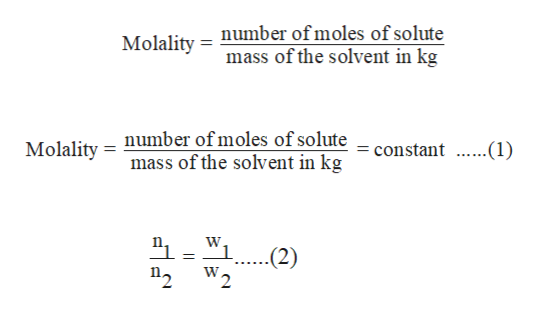# Two students prepare two cyclohexane solutions having the same freezing point. Student 1 uses 26.6 g of cyclohexane solvent, and student 2 uses 24.1 g of cyclohexane solvent. Which student has the greater number of moles of solute?

Question
128 views

Two students prepare two cyclohexane solutions having the same freezing point. Student 1 uses 26.6 g of cyclohexane solvent, and student 2 uses 24.1 g of cyclohexane solvent. Which student has the greater number of moles of solute?

check_circle

Step 1

Given that students use the two solutions of cyclohexane that have same freezing point.

It can be written as,

Here,

ΔTf is the depression in freezing point.

Kf is the molal freezing point depression constant.

m is the molality of the solution.

Depression in freezing point can also be written as follows:

Step 2

The molality of a solution is the ratio of number of moles of solute to that of the weight of the solvent in kg.

Since ΔTf and Kf are constant, molality (m) is also constant.

From above equation (1), it is evident that number of moles of solute is directly proportional to the mass of the solvent.

Mathematically, for the two given solutions it c...help_outlineImage TranscriptioncloseMolality = number of moles of solute mass of the solvent in kg Molality = number of moles of solute mass of the solvent in kg constant 1) W "1. (2) 2W2 fullscreen

### Want to see the full answer?

See Solution

#### Want to see this answer and more?

Solutions are written by subject experts who are available 24/7. Questions are typically answered within 1 hour.*

See Solution
*Response times may vary by subject and question.
Tagged in

### Solutions